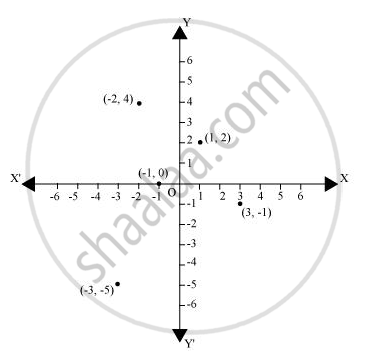Share

# n which quadrant or on which axis do each of the points (– 2, 4), (3, – 1), (– 1, 0), (1, 2) and (– 3, – 5) lie? Verify your answer by locating them on the Cartesian plane. - CBSE Class 9 - Mathematics

ConceptPlotting a Point in the Plane If Its Coordinates Are Given

#### Question

In which quadrant or on which axis do each of the points (– 2, 4), (3, – 1), (– 1, 0), (1, 2) and (– 3, – 5) lie? Verify your answer by locating them on the Cartesian plane.

#### SolutionThe point (-2,4) lies in the IInd quadrant in the Cartesian plane because for point(-2, 4), x-coordinate is negative and y-coordinate is positive.

Again, the point (3, -1) lies in the IVth quadrant in the Cartesian plane because for point(3,-1), x-coordinate is positive and y-coordinate is negative.

The point(-1,0) lies on negative x-axis because for point (-1,0), the value of y-coordinate is zero and the value of x-coordinate is negative.

The point (1,2) lies in the Ist quadrant as for point (1, 2), both x and y are positive.

The point (-3, -5) lies in the IIIrd quadrant in the Cartesian plane because for point (-3, -5), both xand y are negative.

Is there an error in this question or solution?

#### APPEARS IN

NCERT Solution for Mathematics Class 9 (2018 to Current)
Chapter 3: Coordinate Geometry
Ex. 3.30 | Q: 1 | Page no. 65

#### Video TutorialsVIEW ALL 

Solution n which quadrant or on which axis do each of the points (– 2, 4), (3, – 1), (– 1, 0), (1, 2) and (– 3, – 5) lie? Verify your answer by locating them on the Cartesian plane. Concept: Plotting a Point in the Plane If Its Coordinates Are Given.
S